Gravitation homework Help at TutorEye

## Gravitation:

Gravitation or gravity is the force of attraction between particles separated by a definite distance.It is the universal force of attraction acting between all the bodies in the universe. The concept of gravity is a natural phenomenon between two bodies, where each body exerts force on each other. It controls the movement of bodies in the solar system and also determines its trajectories.

## GravitationSample Questions:

Question 1: The equation of Newton’s law of gravitation is dependent on

a) Mass                  b) Gravitational constant

c) Distance            d) All of the above

Answer: d) All of the above

Explanation: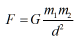Question 2: If the radius of earth decreases by 5%, the new value of gravitational constant G would

a) decrease by 0.5 %              b) increase by 0.5 %

c) remains constant                d) None of the above

Explanation: Universal gravitational constant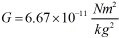Question 3: Which of these is the correct expression for the SI unit of gravitational constant G ?

a)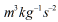b)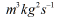c)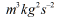d)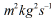Answer: a)Explanation: Force F = ma

Question 4: Two spherical bodies of radius “r” and density “d” are in contact with each other. The gravitational force between them is proportional to

a)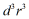b)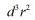c)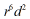d)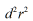Answer: c)Explanation: Mass of body = Volume * Density

Question 5: The value of acceleration due to gravity is least on

a) Poles                                   b) Equator

c) Equator and poles               d) None of the above

Explanation: Acceleration due to gravity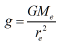Question 6: A body of mass M is split into two masses of (m) and (M - m) What is the relation between two masses so that the force of gravitation is maximum.

a) m = M            b) m = M/3

c) m.M = 2         d) m = M/2

Explanation:  Given,

Question 7: The value of gravitational constant G on the planet Mars is _________ than on Earth.

a) greater            b) smaller

c) same               d) none of the above

Explanation: Gravitational constant is an universal constant and remains constant everywhere

Question 8: A body weighs 10kg on the surface of the earth. How much it will weigh on the planet, whose mass is 1/7 of and radius half of the earth.

a) 20 kg         b) 10 kg

c) 30 kg         d) 50 kg

Explanation: Given,

Question 9: The gravitational force between two identical spheres in contact with each other is proportional to

a)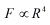b)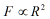c)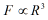d)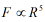Answer: a)Explanation: Given,

Question 10: Gravitational constant is experimentally determined by

a) Galileo’s experiment              b) Pascal’s experiment

c) Newton’s  experiment            d) Cavendish experiment

Explanation: Gravitational constant G